# GLMMs: worked examples

2014-05-06 12:43:33

These are worked examples for a forthcoming book chapter on mixed models (in Ecological Statistics: Contemporary Theory and Application, editors Negrete, Sosa, and Fox).

It may move or be renamed eventually, but for right now the source (.rmd) file and data files are available from http://www.math.mcmaster.ca/bolker/R/misc/foxchapter:

There's a lot of material here, and I have erred slightly on the side of including things, and on the side of compact rather than elementary code.

I use a very large set of packages in this example, because I am trying out a wide range of approaches. You should whittle these down to just the ones you need for any given analysis …

## primary GLMM-fitting packages:
library(lme4)
library(MCMCglmm)
library(blme)
library(MASS)          ## for glmmPQL
library(nlme)          ## for intervals(), tundra example
## auxiliary
library(ggplot2)       ## for pretty plots generally
## ggplot customization:
theme_set(theme_bw())
library(grid)          ## for unit()
zmargin <- theme(panel.margin=unit(0,"lines")) ## to squash facets together ...
library(scales)        ## for squish()
library(gridExtra)     ## for grid.arrange()
library(proto)         ## for horizontal line range plot
source("geom-linerangeh.R")  ## for horizontal line ranges
library(coefplot2) ## coefficient plots
library(coda)      ## MCMC diagnostics
library(aods3)     ## overdispersion diagnostics
library(scapeMCMC) ## pretty plots from MCMC fits
library(bbmle)     ## AICtab
library(pbkrtest)  ## parametric bootstrap
library(Hmisc)
## library(plotrix)  ## for plotCI
## library(emdbook)  ## for curve3d()
## for general-purpose reshaping and data manipulation:
library(reshape2)
library(plyr)
library(numDeriv)


Package versions used (core packages only):

##      lme4  glmmADMB  MCMCglmm      blme  pbkrtest coefplot2      coda
##     1.1.7     0.7.7      2.18     1.0.2     0.3.8   0.1.3.2    0.16.1
##     aods3     bbmle
##     0.4.1    1.0.16


## Tundra carbon

These data were originally analyzed in Belshe et al. 2013, “Tundra ecosystems observed to be CO2 sources due to differential amplification of the carbon cycle”, Ecology Letters 16 (10), 1307-1315 (doi: 10.1111/ele.12164).

### Data exploration

mc1 <- read.csv("tundra.csv",na.strings=c("-","NA"))


A first look at the data, plotting net ecosystem exchange during the growing season (GS.NEE) against year, using colour to distinguish sites, and superimposing a separate linear regresssion (with confidence bands) per site:

ggplot(mc1,aes(x=Year,y=GS.NEE,colour=Site))+geom_point()+
geom_smooth(method="lm",alpha=0.3)+
## oob=squish retains the (truncated) confidence bands;
## otherwise bands that went outside the limits would disappear
## (requires the 'scales' package be loaded)
scale_y_continuous(limits=c(-150,400),oob=squish)+
scale_colour_discrete(guide="none") ## suppress legend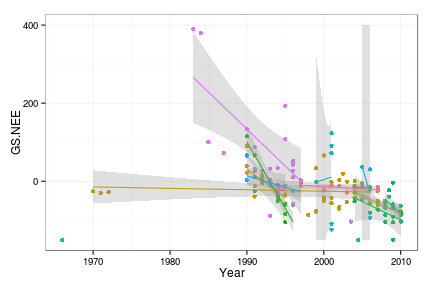We have suppressed the legend for the colours, because there are a lot of sites and their names will not be particularly meaningful to people who are not tundra biologists. There is lots of variability among sites, both in the trend and in the uncertainty of the trend. The uncertainty is caused in part by noisiness of the data, and part by sparsity/shortness of the time series for individual sites.

In some cases there were multiple observations per site in a single year. We could have handled this by adding a year-within-site random variable, but it proved to be easier to aggregate these cases by calculating the mean, and account for the different amount of sampling by weighting site-year combinations according to the number of samples.

The following (rather complicated) function aggregates the data; in the course of our analysis we ended up needing to aggregate several different response variables according to several different sets of grouping variables. We re-centred the year to have year=0 at the beginning of the observation period.

aggfun <- function(dat,agg=c("Year","Site"),response="Winter.adj",
baseYear=min(mc1$Year)) { ## select only site, year, and response sub1 <- na.omit(dat[,c("Site","Year",response)]) ## compute means of non-aggregation variables agg1 <- aggregate(sub1[!names(sub1) %in% agg],by=sub1[agg],FUN=mean) ## compute sample size of non-aggregation variables aggn <- aggregate(sub1[response],by=sub1[agg],FUN=length) names(aggn)[ncol(aggn)] <- "n" ## assumes response is last column ## put mean and sample size together agg2 <- merge(agg1,aggn) ## recompute centred year agg2$cYear <- agg2$Year - baseYear agg2 } mc2 <- aggfun(mc1,response="GS.NEE") ## center year at the mean rather than the date of ## the first observation: mc2B <- aggfun(mc1,response="GS.NEE",baseYear=mean(mc1$Year))


The aggregated data show a similar picture:

ggplot(mc2,aes(x=cYear,y=GS.NEE,colour=Site))+
geom_point(aes(size=n),alpha=0.7)+
geom_smooth(method="lm",alpha=0.3,aes(weight=n))+
scale_y_continuous(limits=c(-150,400),oob=squish)+
scale_colour_discrete(guide="none")+
scale_size_continuous(range=c(2,5),breaks=c(1,3,6))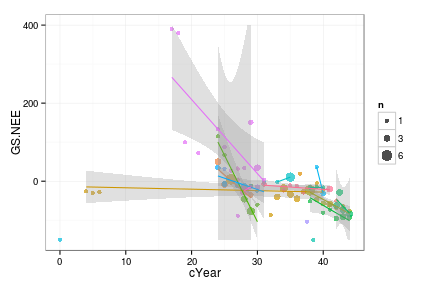### Fitting

We use nlme::lme because at present it is the only easy way to allow for temporal autocorrelation in a LMM in R.

• we use corCAR1, which implements a continuous-time first-order autocorrelation model (i.e. autocorrelation declines exponentially with time), because we have missing values in the data. The more standard discrete-time autocorrelation models (lme offers corAR1 for a first-order model and corARMA for a more general model) don't work with missing data.
• The weights=varFixed(~I(1/n)) specifies that the residual variance for each (aggregated) data point is inversely proportional to the number of samples.
• The control argument lets the model try more iterations (otherwise we get an error).
cmod_lme <- lme(GS.NEE ~ cYear,
data=mc2, method="REML",
random = ~ 1 + cYear | Site,
correlation=corCAR1(form=~cYear|Site),
weights=varFixed(~I(1/n)),
control=list(maxIter=10000, niterEM=10000))


Model summary:

summary(cmod_lme)

## Linear mixed-effects model fit by REML
##  Data: mc2
##     AIC   BIC logLik
##   879.8 896.4 -432.9
##
## Random effects:
##  Formula: ~1 + cYear | Site
##  Structure: General positive-definite, Log-Cholesky parametrization
##             StdDev  Corr
## (Intercept) 147.904 (Intr)
## cYear         4.232 -1
## Residual     60.063
##
## Correlation Structure: Continuous AR(1)
##  Formula: ~cYear | Site
##  Parameter estimate(s):
##    Phi
## 0.4039
## Variance function:
##  Structure: fixed weights
##  Formula: ~I(1/n)
## Fixed effects: GS.NEE ~ cYear
##              Value Std.Error DF t-value p-value
## (Intercept) 108.89     48.02 57   2.267  0.0272
## cYear        -3.85      1.37 57  -2.802  0.0069
##  Correlation:
##       (Intr)
## cYear -0.987
##
## Standardized Within-Group Residuals:
##     Min      Q1     Med      Q3     Max
## -2.7596 -0.4218 -0.1201  0.2685  3.0855
##
## Number of Observations: 82
## Number of Groups: 24


The results generally look sensible: the only warning sign is that the among-site variation in baseline NEE ((Intercept)) and the among-site variation in slope are perfectly correlated (i.e., the -1 term in the Corr column under Random effects). We weren't happy with this, but we kept the full random effects model anyway. The alternative, dropping the random effect of year, seemed unacceptable in our case. Recentring the data at the mean year ($$\approx$$ 1997) improves things slightly:

cmod2_lme <- update(cmod_lme,data=mc2B)


VarCorr() or summary() show that the intercept and slope random effects are still very strongly, but not perfectly, correlated ($$\rho=0.973$$); the fixed-effect intercept is very different (of course), and the year effect is almost identical, but its standard error is larger (so its $$p$$-value doubles).

##              Value Std.Error DF t-value p-value
## (Intercept) -17.42      7.77 57   -2.24   0.029 *
## cYear        -3.84      1.51 57   -2.55   0.014 *
## ---
## Signif. codes:  0 '***' 0.001 '**' 0.01 '*' 0.05 '.' 0.1 ' ' 1


We can also fit the model with lmer from the lme4 package: it's faster and allows for crossed random effects (neither of which really matters here), but unfortunately it can't incorporate temporal autocorrelation in the model:

cmod_lmer <- lmer(GS.NEE ~ cYear + (1+cYear|Site),
data=mc2B, REML=TRUE,
weights=n)
summary(cmod_lmer)

## Linear mixed model fit by REML ['lmerMod']
## Formula: GS.NEE ~ cYear + (1 + cYear | Site)
##    Data: mc2B
## Weights: n
##
## REML criterion at convergence: 874.2
##
## Scaled residuals:
##     Min      1Q  Median      3Q     Max
## -2.9021 -0.3504 -0.0797  0.3015  2.9315
##
## Random effects:
##  Groups   Name        Variance Std.Dev. Corr
##  Site     (Intercept)  116.0   10.77
##           cYear         19.9    4.47    -1.00
##  Residual             3355.3   57.93
## Number of obs: 82, groups:  Site, 24
##
## Fixed effects:
##             Estimate Std. Error t value
## (Intercept)   -16.30       7.34   -2.22
## cYear          -3.75       1.34   -2.79
##
## Correlation of Fixed Effects:
##       (Intr)
## cYear -0.417


Note that weights here are specified as n rather than 1/n (varFixed() in the lme call specifies the variance, rather than the actual weights of different observations)

The results are not too different – the cYear fixed-effect slope is slightly smaller than for the lme fit (-3.75 vs. -3.84 (g C m2 /year/season)/year), but the standard error is smaller, so the $$t$$-statistic is similar (-2.79 vs. -2.55).

We have to admit that the model we are using is just a little bit more complex than the data can easily handle, and especially that Toolik is quite different from the other sites … making the estimates somewhat unstable.

### Diagnostics

Here are the default residuals-vs.-fitted plot; the scale-location plot ($$\sqrt{|\textrm{residual}|}$$ vs. fitted); a plot of the residuals as a function of year; and the Q-Q plot.

colvec <- c("#ff1111","#007eff") ## second colour matches lattice default
grid.arrange(plot(cmod2_lme,type=c("p","smooth")),
plot(cmod2_lme,sqrt(abs(resid(.)))~fitted(.),
col=ifelse(mc2$Site=="Toolik, AK",colvec,colvec), type=c("p","smooth"),ylab=expression(sqrt(abs(resid)))), ## "sqrt(abs(resid(x)))"), plot(cmod2_lme,resid(.,type="pearson")~cYear, type=c("p","smooth")), qqnorm(cmod2_lme,abline=c(0,1), col=ifelse(mc2$Site=="Toolik, AK",colvec,colvec)))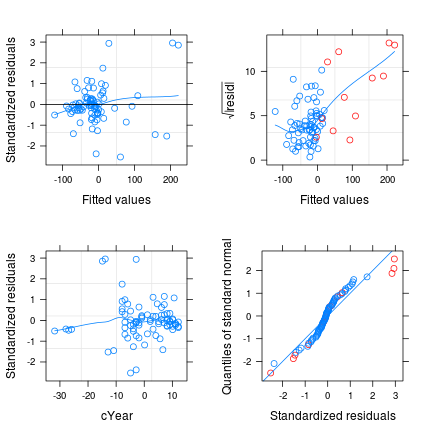The diagnostics look OK – mostly.

• The biggest concern is that the scale-location plot (upper right) shows that the variance increases with the fitted values, which is entirely driven by data from the Toolik site (see below). (I initially diagnosed the problem by using id=0.05, which labels relatively large residuals by site – since they were mostly from Toolik I changed the diagnostic plot above to colour all of the Toolik points differently.) The standard approach would be to transform the data, but transforming data in way that (1) preserves the linear trends in the data (2) allows for negative as well as positive values is a bit challenging (we could try the yjPower() function in the car package). We could also use the weights argument to specify that the variance increases with the fitted values, but we are already using weights to account for the number of samples aggregated per data point …
• The Q-Q plot is a little bit funky, too, also partially driven by Toolik, although non-normality is generally less of a concern than heteroscedasticity.
ggplot(mc2,aes(x=cYear,y=GS.NEE,colour=(Site=="Toolik, AK")))+
geom_point(aes(size=n),alpha=0.7)+
geom_smooth(method="lm",alpha=0.3,aes(weight=n,group=Site))+
scale_colour_discrete(name="Site",breaks=0:1,labels=c("other","Toolik"))+
scale_y_continuous(limits=c(-150,400),oob=squish)+
scale_size_continuous(range=c(2,5),breaks=c(1,3,6))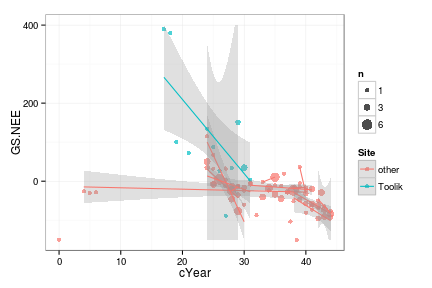We should also check whether our model has dealt with temporal autocorrelation properly. One potential trap when diagnosing autocorrelation in lme models is that the ACF() function by default uses a form of the residuals that do not correct for autocorrelation, even when there is a correlation term specified in the fitted model: you have to use resType="normalized" to get autocorrelation-corrected residuals, as shown in the right-hand plot below:

grid.arrange(plot(ACF(cmod2_lme),alpha=0.05),
plot(ACF(cmod2_lme,resType="normalized"),alpha=0.05),
nrow=1)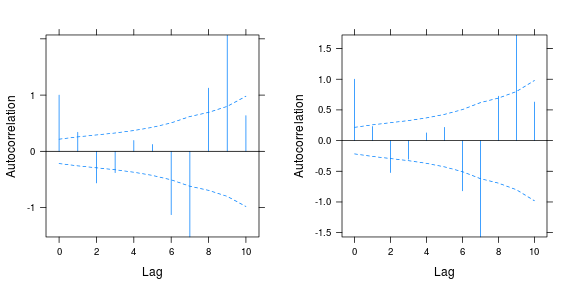The autocorrelation term in the model has successfully corrected for the autocorrelation at lag 1, but there are some large anomalies for longer time lags. These are somewhat worrisome, but autocorrelation estimates this large (absolute value >1) probably represent other anomalies in the data, rather than an actual signal of autocorrelation.

We can try refitting the model without the Toolik data. As it turns out we run into convergence problems and have to drop the autocorrelation structure from the model.

cmod3_lme <- update(cmod2_lme,
data=subset(mc2,Site!="Toolik, AK"),
correlation=NULL)
plot(ACF(cmod3_lme),alpha=0.05) ## not shown, but looks fine


We are again stuck between what the data might tell us to do (throw out the Toolik data), and what we want to do as biologists (incorporate all the data). At least the results (the slope of NEE with respect to time) are not too much different when we fit the model without the Toolik data (the slope is actually steeper, although noisier): the coefficient table from summary(cmod3_lme) looks like this:

##              Value Std.Error DF t-value p-value
## (Intercept) 109.02     50.16 46    2.17   0.035 *
## cYear        -4.42      1.80 46   -2.46   0.018 *
## ---
## Signif. codes:  0 '***' 0.001 '**' 0.01 '*' 0.05 '.' 0.1 ' ' 1


What about the estimated random effects (conditional modes)? They are easy to extract, using the ranef() method, which is the same way you would do it with lme4 or glmmADMB. Plotting them takes a little bit more effort – the default plot produced by the nlme package is OK, but (1) it doesn't plot the random effects in sorted order (which is a good default); (2) it doesn't produce standard errors; (3) it makes it a bit hard if we want to plot only one set of random effects (in this case, since lme has estimated the among-site variance in intercept to be very close to zero, it's not really worth plotting the intercept random effects).

rr <- ranef(cmod2_lme)
rr.order <- rr[order(rr$cYear),] ## order by RE slope value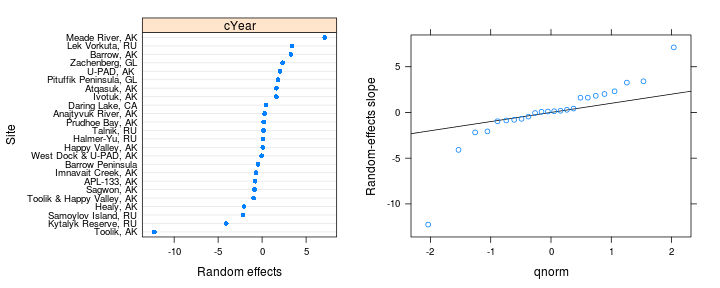We can more easily plot the random effects for the lmer fit (we also get confidence intervals without too much fuss): dotplot(ranef(cmod_lmer,condVar=TRUE), lattice.options=list(layout=c(1,2)))  ##$Site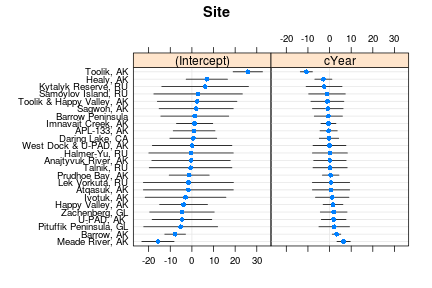• In contrast to the lme fit, the lmer fit gives a non-negligible estimate for the among-site standard deviation.
• You can use qqmath() instead of dotplot() to get Q-Q plots of the conditional modes.
• Toolik still stands out as an outlier.

### Inference

Looking more carefully at the model fit, we can see that the intercept variation has disappeared – this means that, at the temporal center of the data, the variation among sites is essentially indistinguishable from measurement error. There is still substantial variation in the slope, along with the almost-perfect correlation described above:

VarCorr(cmod2_lme)

## Site = pdLogChol(1 + cYear)
##             Variance  StdDev    Corr
## (Intercept) 4.440e-13 6.664e-07 (Intr)
## cYear       2.579e+01 5.079e+00 0.973
## Residual    3.469e+03 5.890e+01

##              Value Std.Error DF t-value p-value
## (Intercept) -17.42      7.77 57   -2.24   0.029 *
## cYear        -3.84      1.51 57   -2.55   0.014 *
## ---
## Signif. codes:  0 '***' 0.001 '**' 0.01 '*' 0.05 '.' 0.1 ' ' 1


Because the model only has a single continuous predictor, the conclusions of summary(), drop1(), and anova() are all equivalent (the anova method for lme has a useful type="marginal" option, but you should be very careful when using it with models containing interactions – car::Anova is safer). Note that lme gets the degrees-of-freedom calculation wrong for random-slopes models: because the year effect varies among sites, it should be tested with 23 denominator df rather than 53 as reported by lme. However, it doesn't make a huge difference here:

fstat <- anova(cmod2_lme)["cYear","F-value"]
pf(fstat,1,53,lower.tail=FALSE)  ## as reported by lme

##  0.01387

pf(fstat,1,23,lower.tail=FALSE)  ## correct

##  0.01809


In Belshe et al. we reported that when we simulated data according to the null hypothesis (incorporating information about the variation in intercepts and slopes across sites, and autocorrelation, but with the population-level slope set to zero), we saw an inflated type I error. Therefore, we used this parametric bootstrap distribution of the $$t$$ statistic to test the fixed effect of slope, which approximately doubled our $$p$$-value.

The nlme package uses intervals(), rather than confint(), to extract confidence intervals: if we try intervals(cmod2_lme) we get an error about “non-positive definite approximate variance-covariance” (which should be yet another indication that our model is somewhat unstable or overfitted). However, we can work around this by restricting our question to the fixed effects only:

intervals(cmod2_lme,which="fixed")

## Approximate 95% confidence intervals
##
##  Fixed effects:
##               lower    est.   upper
## (Intercept) -32.966 -17.416 -1.8663
## cYear        -6.856  -3.837 -0.8183
## attr(,"label")
##  "Fixed effects:"


We can do a little bit better with the lmer fit, although we still get a warning:

pp <- profile(cmod_lmer)

## Warning: non-monotonic profile

(c_ci <- confint(pp))

##               2.5 %  97.5 %
## .sig01        0.000  29.205
## .sig02       -1.000   1.000
## .sig03        4.324  10.485
## .sigma       35.704  50.654
## (Intercept) -25.400 -18.963
## cYear        -5.050  -3.544


We can also plot the profiles: the fixed-effect parameter profiles look a bit suspicious:

ggplot(as.data.frame(pp),aes(.focal,.zeta))+
geom_point()+geom_line()+
facet_wrap(~.par,scale="free_x")+
geom_hline(yintercept=0,colour="gray")+
geom_hline(yintercept=c(-1.96,1.96),linetype=2,
colour="gray")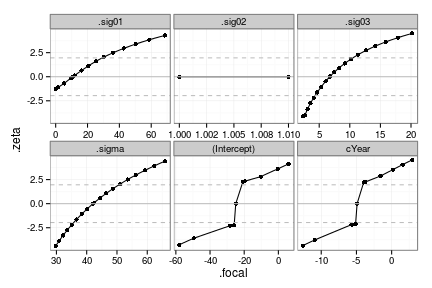## Culcita

The data are from McKeon et al., Multiple defender effects: synergistic coral defense by mutualist crustaceans, Oecologia, 169(4):1095-1103, 2012.

### Data exploration

The basic data can be reduced, for the purposes of this exercise, to a single treatment (ttt) [which consists of combinations of different symbionts: crab, shrimp, both or neither]; a binary response (predation); and a blocking factor (block).

load("culcita.RData")
summary(culcita_dat)

##      block      predation         ttt
##  1      : 8   Min.   :0.000   none  :20
##  2      : 8   1st Qu.:0.000   crabs :20
##  3      : 8   Median :1.000   shrimp:20
##  4      : 8   Mean   :0.625   both  :20
##  5      : 8   3rd Qu.:1.000
##  6      : 8   Max.   :1.000
##  (Other):32


Confirm that this is a randomized block design with 2 replications per treatment per block:

with(culcita_dat,table(ttt,block))

##         block
## ttt      1 2 3 4 5 6 7 8 9 10
##   none   2 2 2 2 2 2 2 2 2  2
##   crabs  2 2 2 2 2 2 2 2 2  2
##   shrimp 2 2 2 2 2 2 2 2 2  2
##   both   2 2 2 2 2 2 2 2 2  2


(the ftable() function can be handy for displaying experimental designs with more than two levels).

Plot summary statistics (mean and bootstrap 95% CI) for treatments, ignoring block structure:

ggplot(culcita_dat,aes(x=ttt,y=predation))+
stat_summary(fun.data=mean_cl_boot,size=2)+
ylim(c(0,1))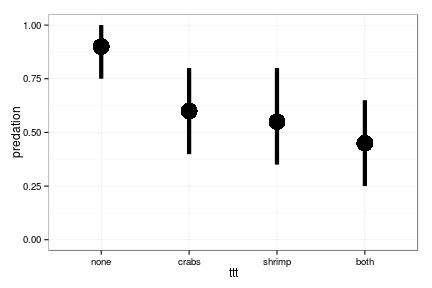The basic conclusion is that symbionts have a definite protective effect; the combination of two symbionts seems slightly more protective than a single symbiont. However, we have to see if this holds up when we account for among-plot variation.

I have yet to find a good way to visualize these data that displays the among-block variation. This is my best attempt, but it's more artistic than compelling:

ggplot(culcita_dat,aes(x=ttt,y=predation,colour=block,group=block))+
stat_summary(fun.y=sum,geom="line",alpha=0.4)+
stat_summary(fun.y=sum,geom="point",alpha=0.7,
position=position_dodge(width=0.25))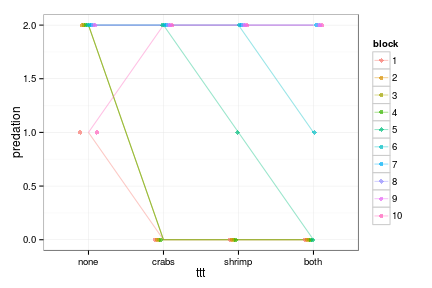### Fitting

First, fit the model in each framework.

#### lme4::glmer

(The syntax pkg::fun refers in general to a function fun in the R package pkg.)

cmod_lme4_L <- glmer(predation~ttt+(1|block),data=culcita_dat,
family=binomial)


Take a quick look at the results:

print(summary(cmod_lme4_L),correlation=FALSE)

## Generalized linear mixed model fit by maximum likelihood (Laplace
##   Approximation) [glmerMod]
##  Family: binomial  ( logit )
## Formula: predation ~ ttt + (1 | block)
##    Data: culcita_dat
##
##      AIC      BIC   logLik deviance df.resid
##     70.7     82.6    -30.4     60.7       75
##
## Scaled residuals:
##     Min      1Q  Median      3Q     Max
## -23.208  -0.173   0.098   0.298   0.894
##
## Random effects:
##  Groups Name        Variance Std.Dev.
##  block  (Intercept) 11.8     3.44
## Number of obs: 80, groups:  block, 10
##
## Fixed effects:
##             Estimate Std. Error z value Pr(>|z|)
## (Intercept)     5.10       1.81    2.81   0.0049 **
## tttcrabs       -3.84       1.46   -2.62   0.0087 **
## tttshrimp      -4.43       1.55   -2.86   0.0043 **
## tttboth        -5.60       1.72   -3.25   0.0012 **
## ---
## Signif. codes:  0 '***' 0.001 '**' 0.01 '*' 0.05 '.' 0.1 ' ' 1


It would be nice to fit the model with a random effect of treatments across blocks as well, but it takes a long time and warns that it has failed to converge …

cmod_lme4_block <- update(cmod_lme4_L,.~ttt+(ttt|block))

## Warning: failure to converge in 10000 evaluations
## Warning: Model failed to converge with max|relative grad| = 122.885 (tol = 0.001, component 2)
## Warning: Model is nearly unidentifiable: large eigenvalue ratio
##  - Rescale variables?


(we could extend the maximum number of iterations by using (e.g.) control=glmerControl(optCtrl=list(maxfun=1e5)), but it probably wouldn't help). (When you use update() you normally specify which variables to add (e.g. .~.+new_var) or subtract (.~.-dropped_var). Here I replaced the entire right-hand side of the formula: I might have been able to replace the intercept-only random effect with a random effect of treatment by subtracting and adding, i.e. .~.-(1|block)+(ttt|block), but I wasn't sure it would work …)

If we examine the parameter estimates from this fit, we see various additional indications of trouble. The crab parameter and several of the variance-covariance parameters are very large and some of the variance-covariance parameters are very strongly correlated with each other, both indications that the model is overfitted:

fixef(cmod_lme4_block)

## (Intercept)    tttcrabs   tttshrimp     tttboth
##       2.221      20.547      -1.377      -4.728

VarCorr(cmod_lme4_block)

##  Groups Name        Std.Dev. Corr
##  block  (Intercept)  0.227
##         tttcrabs    53.559   -0.30
##         tttshrimp    4.385   -0.21  0.99
##         tttboth     23.482   -0.19  0.99  1.00


We can also use model comparison to see that it's not worth adding the extra terms:

AICtab(cmod_lme4_L,cmod_lme4_block,nobs=nrow(culcita_dat))

##                 dAIC df
## cmod_lme4_L      0.0 5
## cmod_lme4_block  0.4 14


(If we use AICc instead of AIC cmod_lme4_block does even worse, by 6 AICc units …)

cmod_lme4_AGQ10 <- update(cmod_lme4_L,nAGQ=10)


The next plot (showing the values of each parameter as a function of the number of quadrature points) shows that using more quadrature points does have some effect, but on an absolute scale the parameters don't vary much: probably the biggest effect is in the estimated among-block standard deviation, increasing irregularly from 3.44 for the Laplace approximation ($$n=1$$) to an asymptote of 3.51.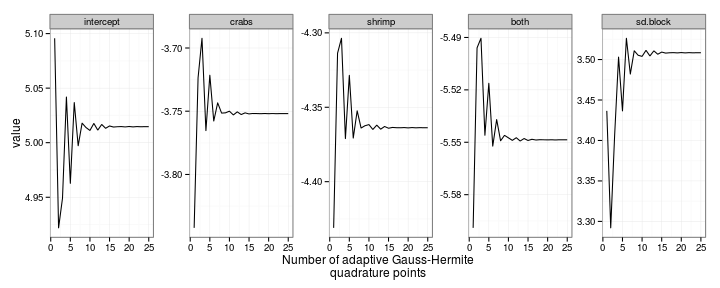The only difference in moving from lme4 to glmmADMB is that you have to express the family argument as a quoted string (i.e., family="binomial" rather than family=binomial):

cmod_gA_L <- glmmadmb(predation~ttt+(1|block),data=culcita_dat,
family="binomial")


The summary() for glmmADMB is nearly identical.

summary(cmod_gA_L)

##
## Call:
## glmmadmb(formula = predation ~ ttt + (1 | block), data = culcita_dat,
##     family = "binomial")
##
## AIC: 70.7
##
## Coefficients:
##             Estimate Std. Error z value Pr(>|z|)
## (Intercept)     5.10       1.81    2.81   0.0049 **
## tttcrabs       -3.84       1.47   -2.62   0.0087 **
## tttshrimp      -4.43       1.55   -2.86   0.0043 **
## tttboth        -5.60       1.73   -3.25   0.0012 **
## ---
## Signif. codes:  0 '***' 0.001 '**' 0.01 '*' 0.05 '.' 0.1 ' ' 1
##
## Number of observations: total=80, block=10
## Random effect variance(s):
## Group=block
##             Variance StdDev
## (Intercept)    11.89  3.448
##
##
## Log-likelihood: -30.35


#### MCMCglmm

The MCMCglmm random effect specification is a little different from glmer and glmmadmb: other differences include

• the Bernoulli (binomial with $$N=1$$) response is specified as family="categorical" (you would code a binomial response with $$N>1$$ as a two-column matrix of successes and failures, as in glm(), and specify family="multinomial2")
• After looking at the diagnostics on the default fit (see below), and with advice from Jarrod Hadfield (the author of MCMCglmm), I decided to
• Set priors.
• The standard way to set priors for the variance-covariance matrices is to specify them as inverse-Wishart distributions; the corresponding Wikipedia page explains that for a single variance, the inverse-Wishart reduces to an inverse-gamma distribution with shape parameter $$\alpha$$ equal to half the Wishart shape ($$\nu$$) parameter. (The inverse-gamma distribution is in turn a distribution of a random variable $$X$$ where $$1/X$$ is Gamma-distributed …) I initially used an informative prior with G=list(list(V=11,nu=1)))) (i.e. specify that the mean of the variance is approximately equal to the among-block variance we got from glmer/glmmADMB and, the shape parameter is 1 (weak)).
• Hadfield suggested that I could instead “use parameter expanded priors which can be made approximately flat (but still proper) for the standard deviation [see prior.c below]. Transforming the variance parameters to the intra-class correlation (ICC) can cause problems however, as it puts high prior mass on extreme ICC's. Parameter expanded priors can also improve mixing considerably for the variances that are close to zero.”
• For the observation-level variance (R) I initially used a strong Gamma-distributed prior with mean 1 and a shape parameter of 10, i.e. $$1/\sigma^2$$ was Gamma-distributed with a mean of 1 and coefficient of variance $$1/\sqrt{5}$$, but Hadfield suggested instead that instead I should simply fix R (which is unidentifiable anyway for binomial trials with a single observation) to 1.
• Specify the number of iterations (nitt), number of iterations to discard (burnin), and thinning (thin) manually, to get a much longer chain than the defaults (nitt=13000, thin=10, burnin=3000).

First attempts, first with everything set to the default and then with a longer run: ⌛ (60 seconds: in general I will use this hourglass icon to indicate code that might take a long time to run – you might want to skip running these chunks.)

cmod_MG0 <- MCMCglmm(predation~ttt,
random=~block,data=culcita_dat,
family="categorical",
verbose=FALSE)
cmod_MG1 <- MCMCglmm(predation~ttt,
random=~block,data=culcita_dat,
family="categorical",verbose=FALSE,
nitt=5e5,burnin=5e4,thin=100)


As we will see below, simply running for longer won't fix everything. Instead, we set stronger/better priors as described above:

prior.c <- list(R=list(V=1,fix=1),
G=list(G1=list(V=1, nu=1, alpha.mu=0, alpha.V=1000)))
cmod_MG <- MCMCglmm(predation~ttt,
random=~block,data=culcita_dat,
slice=TRUE, ## slice sampling: for binary trials
## with independent residuals
pl=TRUE,    ## save posterior distributions of latent
## variables (= conditional modes)
prior=prior.c,
family="categorical",verbose=FALSE,
nitt=13000*10,burnin=3000*10,thin=5*10)

(cmod_MGsum <- summary(cmod_MG))

##
##  Iterations = 30001:129951
##  Thinning interval  = 50
##  Sample size  = 2000
##
##  DIC: 52.33
##
##  G-structure:  ~block
##
##       post.mean l-95% CI u-95% CI eff.samp
## block      59.3     5.03      174      106
##
##  R-structure:  ~units
##
##       post.mean l-95% CI u-95% CI eff.samp
## units         1        1        1        0
##
##  Location effects: predation ~ ttt
##
##             post.mean l-95% CI u-95% CI eff.samp  pMCMC
## (Intercept)      7.28     1.84    13.84      464  0.012 *
## tttcrabs        -5.11    -8.96    -1.58      670  0.002 **
## tttshrimp       -6.00   -10.53    -2.50      387 <5e-04 ***
## tttboth         -7.80   -12.56    -3.76      353 <5e-04 ***
## ---
## Signif. codes:  0 '***' 0.001 '**' 0.01 '*' 0.05 '.' 0.1 ' ' 1


Take note of the eff.samp columns in the summary output, which describe the size of the sample we have taken from the posterior distribution corrected for autocorrelation; values much smaller than the nominal sample size (listed in the third line of the summary) indicate poor mixing.

### Diagnostics and model summaries

#### lme4

Diagnostic plots:

p1 <- plot(cmod_lme4_L,id=0.05,idLabels=~.obs)
p2 <- plot(cmod_lme4_L,ylim=c(-1.5,1),type=c("p","smooth"))
grid.arrange(p1,p2,nrow=1)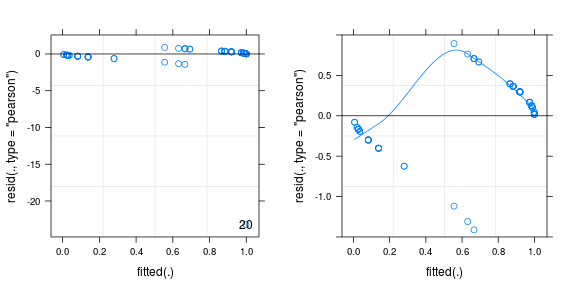The only thing that the default (left-hand) diagnostic plot tells us is that observation #20 has a (very) extreme residual (we use idLabels=~.obs to get outliers labelled by their observation number; otherwise, by default, they are labelled by their groups); if we use ylim=c(-1.5,1) to limit the $$y$$-range, we get (on the right) the usual not-very-informative residuals plot expected from binary data.

### Digression: complete separation

If we exclude observation 20, we see the symptoms of complete separation (e.g., parameter values with $$|\beta|>10$$, huge Wald confidence intervals and large Wald $$p$$-values).

We refit the model with the new data …

newdat <- subset(culcita_dat,
abs(resid(cmod_lme4_L,"pearson"))<10)
cmod_lme4_L2 <- update(cmod_lme4_L,data=newdat)


We can use bglmer from the blme package to impose zero-mean Normal priors on the fixed effects (a 4 $$\times$$ 4 diagonal matrix with diagonal elements equal to 9, for variances of 9 or standard deviations of 3), or add a B element to the priors list for MCMCglmm to specify the same priors:

cmod_blme_L2 <- bglmer(predation~ttt+(1|block),data=newdat,
family=binomial,
fixef.prior = normal(cov = diag(9,4)))
cmod_MG2 <- MCMCglmm(predation~ttt,
random=~block,data=newdat,
family="categorical",verbose=FALSE,
nitt=13e4,burnin=3e4,thin=50,
prior=c(prior.c,
list(B=list(mu=rep(0,4),
V=diag(9,4)))))


Both methods of constraining the parameters give similar results: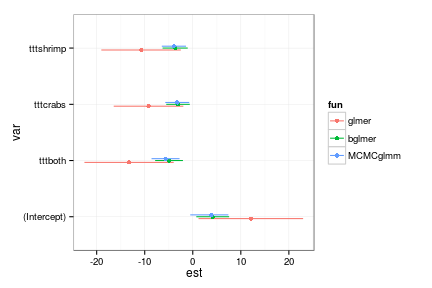There are other packages in R (brglm, logistf) that can handle completely separated data, but they only apply to logistic regression, and they cannot simultaneously incorporate random effects (Pasch et al., American Naturalist 2013 used brglm to handle completely separated data, arguing that random effects were not very important in their system.)

(End of digression)

We can do a little bit better with boxplots grouped by treatment (again limiting the range to exclude the outlier).

plot(cmod_lme4_L,ttt~resid(.,type="pearson"),xlim=c(-1.5,1))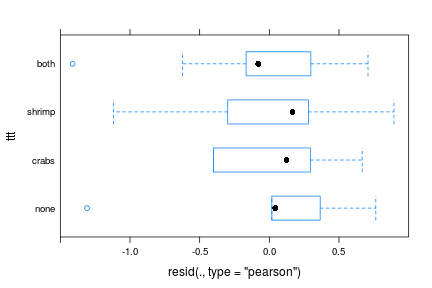Check the random effects:

dotplot(ranef(cmod_lme4_L,condVar=TRUE))

## $block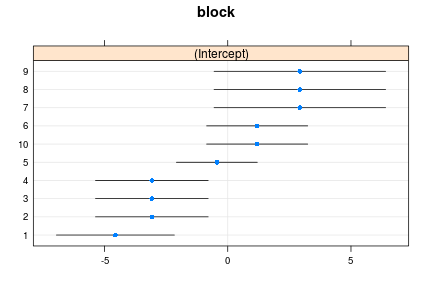There are only a few unique values of the random effects because there are only a few possible configurations per block of predation/no-predation in each treatment. It's worth checking methods(class="merMod") to see all the things you can do with the fit (some of them may be a bit obscure …) The most important are: • fixef() to extract the vector of fixed-effect parameters (confusingly, coef() – which is the accessor method for finding coefficients for most other models in R – gives a matrix showing the estimated coefficients for each block (incorporating the random effects), which I don't find useful very often) • coef(summary(.)) to extract just the table of estimates with standard errors, $$z$$ values, and $$p$$-values • VarCorr() to extract the estimates of the random effect variances and covariances. (Use unlist(VarCorr(.)) if you want the variances as a vector.) • ranef() to extract estimates of the random effects, and dotplot(ranef(.,condVar=TRUE)) or qqmath(ranef(.,condVar=TRUE)) to explore them graphically • plot() for diagnostic plots • AIC(), anova(), drop1(), confint(), profile() for various statistical tests • predict() for predictions; simulate() for simulations based on the model #### glmmADMB The methods and diagnostics for glmmADMB are similar, although not quite as well developed. You can still create the same kinds of diagnostic plots (I have done this one with ggplot2 rather than lattice): augDat <- data.frame(culcita_dat,resid=residuals(cmod_gA_L,type="pearson"), fitted=fitted(cmod_gA_L)) ggplot(augDat,aes(x=ttt,y=resid))+geom_boxplot()+coord_flip()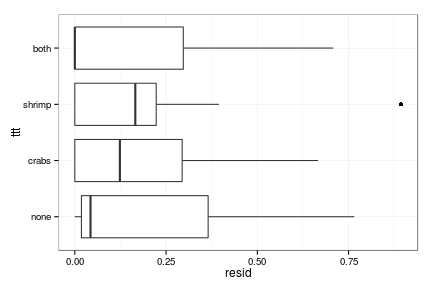#### MCMCglmm Within an MCMCglmm fit the chains are stored in two separate matrices (mcmc objects, actually, but these can be treated a lot like matrices) called Sol (fixed effects) and VCV (variances and covariances). (Random effects are not saved unless you set pr=TRUE.) allChains <- as.mcmc(cbind(cmod_MG0$Sol,cmod_MG0$VCV))  The trace plots for the default parameters: plotTrace(allChains)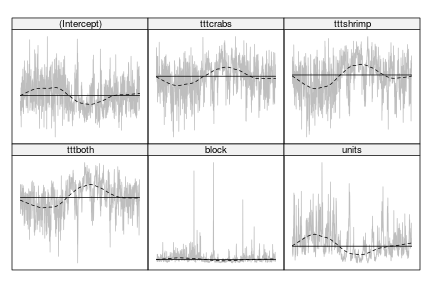(plotTrace is from the scapeMCMC package, and is slightly prettier than the default trace plot you get from xyplot(allChains), or the trace+density plot you get from plot(allChains), but they all show the same information.) This trace plot is terrible: it indicates at the very least that we need a longer burn-in period (to discard the transient) and a longer chain with more thinning (to make the chains look more like white noise). It's also worrying that the units variance appears to be get stuck near zero (although here the problem may be with the large transient spikes distorting the scale and making the rest of the chain look non-variable). Running longer: allChains2 <- as.mcmc(cbind(cmod_MG1$Sol,cmod_MG1$VCV)) plotTrace(allChains2,axes=TRUE,las=1)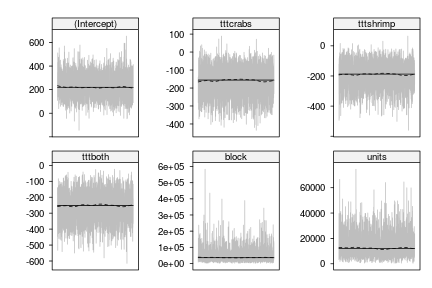This looks OK at first glance, but the magnitudes of the parameters (suppressed by default from the tracePlot results since we're initially interested only in the pattern, not the magnitude, of the parameters) suggest a problem: the values of the fixed-effect parameters are in the hundreds, when any values with $$|\beta|>10$$ suggest a problem. The fundamental problem here is that, for both technical and philosophical reasons, MCMCglmm always adds an observation-level variance (referred to in MCMCglmm as the “R-structure”, for “residual structure”), corresponding to an overdispersion term. For binary data, $\text{logit}(p_i) = m_i + \epsilon_i$ where $$\epsilon_i \sim N(0,\sigma^2)$$ then the expected value of $$p_i$$ is the average of $$\text{logistic}(m_i+\epsilon_i)$$. This is a nonlinear average, and so the mean of $$p_i$$ is not equal to $$\text{logistic}(m_i)$$ (this phenomenon is called Jensen's inequality; Ruel and Ayres 1999 give an ecologist-friendly explanation). If $$\ell(x)=1/(1+\exp(-x))$$ is the logistic function then the mean of $$p_i$$ is approximately $\overline{p_i} \approx \ell(m_i) + \left.\frac{d^2\ell}{dx^2}\right|_{x=m_i} \cdot \sigma^2/2$ We won't bother to show the math of $$d^2\ell(x)/dx^2$$ here: suffice it to say that this term varies from about +0.1 to -0.1 depending on the baseline value $$m_i$$, so that the estimates of $$m_i$$ and the observation-level variance $$\sigma^2$$ are confounded:In the MCMC example here, the observation-level variance has drifted to a very large value ($$\sigma^2_R \approx$$ 1.35 × 104), and the initial (variance-free) value of the baseline predation probability was low, so the variance inflates the mean by a great deal and the intercept parameter becomes strongly negative to compensate. To deal with the confounding between these variables, we fix the value of $$\sigma^2_R$$ as described above. The results: allChains3 <- as.mcmc(cbind(cmod_MG$Sol,cmod_MG$VCV)) ## units variance is fixed to 1 anyway, we don't need ## to examine it allChains3 <- allChains3[,colnames(allChains3)!="units"] plotTrace(allChains3,axes=TRUE,las=1)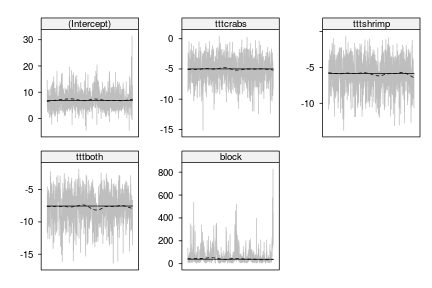Now the magnitudes of the parameters look a little bit more sensible. The chains for the variances are a little bit “spiky” because the posterior densities have long right tails, so it can be helpful to look at them on a log scale (it's easier to see the details when the distribution is symmetric): vcChain <- log10(cmod_MG$VCV)[,1]
plotTrace(vcChain)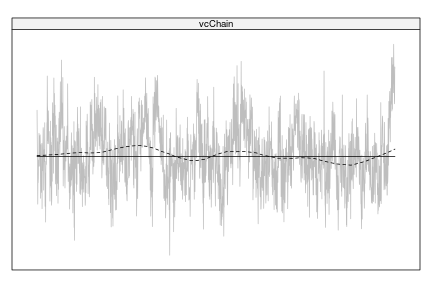The block variance is still slightly worrying: the trace plot still shows some pattern (we want it to be more or less indistinguishable from white noise), and the effective sample size (106) is much smaller than our nominal sample size of (see the eff.samp column in the summary above).

We have one more thing to worry about. If we extract cmod_MG$Liab (the conditional modes/latent variables) and plot their histogram: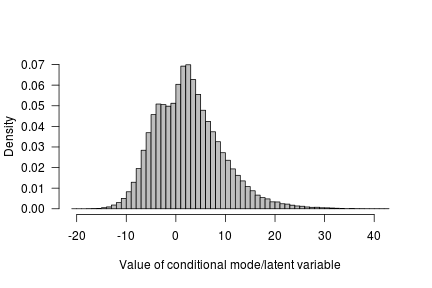Some of the latent variables are larger than 20. The likelihood of the linear predictor is essentially flat from $$[20,\infty]$$. If this happens for all observations belonging to a single term (fixed or random) then there is nothing constraining that term except the prior ($$B$$) or for random effects the posterior variance. Consequently issues can arise, without suitable prior or hyper prior specifications – this suggests that we might want to consider using the slightly stronger priors on $$B$$ specified above when we were trying to deal with complete separation. In order to compare the estimates we get from MCMCglmm with the other models – which do not incorporate an observation-level random effect – we Rescale block variance to what it would be had we set the units variance to zero, and overlay lmer estimate: ## see eqs. 2.13, 2.14 of the MCMCglmm 'CourseNotes' vignette ## for further information & references: c2 <- ((16 * sqrt(3))/(15 * pi))^2 cmod_MGsc <- cmod_MG cmod_MGsc$VCV[,"block"] <-
cmod_MGsc$VCV[,"block"]/(1+c2*cmod_MGsc$VCV[,"units"])
cmod_MGsc$Sol[] <- cmod_MGsc$Sol/sqrt(1+c2*cmod_MGsc$VCV[,"units"]) cmod_MGsc$VCV[,1] <- sqrt(cmod_MGsc$VCV[,1])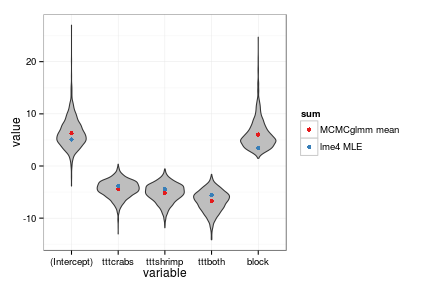The modes of the MCMCglmm results (the fattest part of the “violins”) agree well with the lme4 MLEs, for the most part: the lme4 estimate of the among-block standard deviation is a bit lower than the MCMCglmm estimate. (When we display the overall parameter comparison below, we will show the MCMCglmm means instead.) ### Inference We get the values of the parameters and the (Wald) standard errors, $$Z$$- and $$p$$-values (for glmer() and glmmADMB()), or the (quantile-based) credible intervals and “pMCMC” values from the summary() output, as shown above. If we want more accurate profile confidence interval, parametric bootstrap, or MCMC-based confidence intervals for glmer()- or glmmADMB()-based analyses, we use confint(): ⌛ Compute profile confidence intervals: cmod_lme4_L_CI_prof <- confint(cmod_lme4_L) cmod_lme4_L_CI_quad <- confint(cmod_lme4_L,method="Wald") cmod_lme4_L_CI_boot <- confint(cmod_lme4_L,method="boot")  The parameter estimates and confidence intervals are reasonably consistent across packages and algorithms. • glmer and glmmADMB give the same point estimates – reassuringly, since they are both using (different implementations of) the Laplace approximation method. MCMCglmm gives slightly lower (= more negative) estimates of the point estimates, and higher estimates of the among-block standard deviation; this is in part because MCMCglmm is reporting the posterior mean, which for a distribution with a long right tail is larger than the posterior mode (analogous to the maximum likelihood estimate). • the likelihood profile and Wald CIs are similar (glmmADMB's Wald intervals are slightly wider than glmer's, because glmer's calculation does not account for uncertainty due to the random-effects parameters; this is not really obvious from the plot). • The MCMCglmm confidence intervals are a bit wider, and the parametric bootstrap confidence intervals are extremely wide (they're truncated at $$\pm 15$$ for display purposes). For some parametric bootstrap realizations, the simulated predation outcomes are such that complete separation occurs (some blocks, or some treatments, are either always or never attacked by predators), leading to infinite estimates of the parameters (log-odds differences). This is not a big practical difficulty because the direction of the uncertainty is toward more extreme results …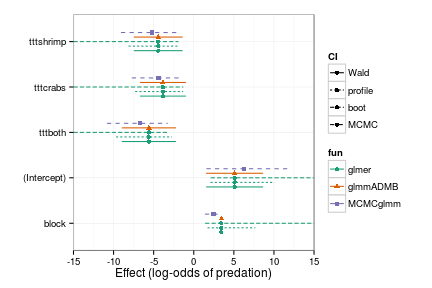### Model comparison/hypothesis testing The summary of the model gives us crude (Wald) estimates of the $$p$$-values for each individual parameter: coef(summary(cmod_lme4_L))  ## Estimate Std. Error z value Pr(>|z|) ## (Intercept) 5.096 1.811 2.814 0.004899 ## tttcrabs -3.842 1.465 -2.623 0.008710 ## tttshrimp -4.431 1.551 -2.856 0.004290 ## tttboth -5.599 1.724 -3.247 0.001165  We can get the overall $$p$$-value for the effect of treatment and use anova() to do a likelihood ratio test: cmod_lme4_0 <- update(cmod_lme4_L,.~.-ttt) anova(cmod_lme4_L,cmod_lme4_0)  ## Data: culcita_dat ## Models: ## cmod_lme4_0: predation ~ (1 | block) ## cmod_lme4_L: predation ~ ttt + (1 | block) ## Df AIC BIC logLik deviance Chisq Chi Df Pr(>Chisq) ## cmod_lme4_0 2 86.2 91.0 -41.1 82.2 ## cmod_lme4_L 5 70.7 82.6 -30.4 60.7 21.5 3 8.3e-05 *** ## --- ## Signif. codes: 0 '***' 0.001 '**' 0.01 '*' 0.05 '.' 0.1 ' ' 1  We could also use drop1(cmod_lme4_L,test="Chisq") to refit the reduced model automatically (helpful if we have a more complex model with more separate fixed effects to test). We can also get parametric bootstrap confidence intervals (). I initially tried to do this via PBmodcomp(cmod_lme4_L,cmod_lme4_0,nsim=400), but failed when one of the model-refitting steps produced an error. Instead, I defined my own parametric bootstrap function which tests to make sure that neither of the refitting steps failed: PBsimfun <- function(m0,m1,x=NULL) { if (is.null(x)) x <- simulate(m0) m0r <- try(refit(m0,x[]),silent=TRUE) if (inherits(m0r,"try-error")) return(NA) m1r <- try(refit(m1,x[]),silent=TRUE) if (inherits(m1r,"try-error")) return(NA) c(-2*(logLik(m0r)-logLik(m1r))) }  set.seed(101) PBrefdist <- replicate(400,PBsimfun(cmod_lme4_0,cmod_lme4_L))  Out of 400 replicates, 4 are NA (the refitting failed somehow), and 1 is <0 by a tiny amount (numerical inaccuracy in the fitting). It is also appropriate to add the observed value of the difference in $$-2 \log(L)$$ to the reference distribution: obsval <- -2*(logLik(cmod_lme4_0)-logLik(cmod_lme4_L)) PBrefdist <- c(na.omit(pmax(PBrefdist,0)),obsval)  The histogram looks pretty close to the expected $$\chi^2_3$$ distribution for the likelihood ratio test (3 degrees of freedom because we are comparing a model with 4 vs. 1 fixed-effect parameters):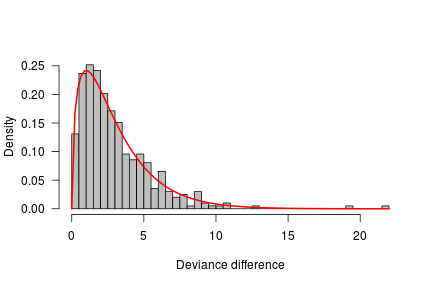However, we would probably prefer a real $$p$$-value, which we get by computing the fraction of values in the reference distribution >= the observed value (we can use mean(ref>obs) as a shortcut to compute this proportion). Since the observed value is included in the reference distribution, the $$p$$-value can never be less than $$1/(\mbox{nsim}+1)$$, which is its value in this case: mean(PBrefdist>=obsval)  ##  0.002519  If we want to use parametric bootstrapping to test a different hypothesis, either based on the assumption that a single parameter is zero or based on some particular contrast among the treatments (e.g. that the effect of two symbionts is equal to the effect of either symbiont alone), we have to set up our own dummy variables rather than relying on R's automatic handling of categorical (factor) variables. Suppose we want to compare the effect of a single symbiont of either kind to the effect of two symbionts (i.e., the average of crabs and shrimp). This is a bit more challenging than testing the overall effect of treatment. First we have to set up an appropriate contrast to partition the effects of treatment. In this case we change the default treatment contrasts (control, control vs. crab, control vs. shrimp, control vs. both) to test (i) control, (ii) any symbionts vs no symbionts, (iii) crabs alone vs shrimp alone, (iv) one symbiont vs two symbionts. invcontr <- matrix(c(1,0,0,0, -1,1/3,1/3,1/3, 0,-1,1,0, 0,-1/2,-1/2,1), byrow=TRUE,ncol=4, dimnames=list(c("none","symbiont", "C_vs_S","1_vs_2"), levels(culcita_dat$ttt)))
cmat0 <- solve(invcontr)[,-1]


Because we will want to do a parametric bootstrap test of one particular contrast, we will need to be able to exclude that variable from the model. We do this by setting up the model matrix explicitly and using its columns explicitly as numeric dummy variables:

X <- model.matrix(~ttt,
data=culcita_dat,
contrasts=list(ttt=cmat0))
culcita_dat2 <- with(culcita_dat,data.frame(block,predation,
X[,-1]))
cmod_lme4C_L <- glmer(predation~tttsymbiont+
tttC_vs_S+ttt1_vs_2+
(1|block),data=culcita_dat2,
family=binomial)
## fit reduced model as well
cmod_lme4C_L0 <- update(cmod_lme4C_L,.~.-ttt1_vs_2)


Now we do the parametric bootstrap comparison (⌛!):

set.seed(101)
PBrefdist2 <- replicate(400,PBsimfun(cmod_lme4C_L0,cmod_lme4C_L))


And compute the $$p$$-value by comparing against the observed value as above:

obsval2 <- -2*(logLik(cmod_lme4C_L0)-logLik(cmod_lme4C_L))
PBrefdist2 <- c(na.omit(pmax(PBrefdist2,0)),obsval2)
mean(PBrefdist2>=obsval2)

##  0.1544


Ironically, after all that computational effort this $$p$$-value is nearly identical to the value from the Wald test:

coef(summary(cmod_lme4C_L))

##             Estimate Std. Error z value Pr(>|z|)
## (Intercept)   5.0957      1.811  2.8136 0.004899
## tttsymbiont  -4.6241      1.440 -3.2106 0.001325
## tttC_vs_S    -0.5888      1.099 -0.5357 0.592141
## ttt1_vs_2    -1.4624      1.023 -1.4290 0.153012


### Prediction

Getting predicted values from an lme4 model (or an MCMCglmm model) is fairly straightforward: in this case by specifying re.form=NA we're saying that we want the population-level prediction, i.e. setting the random effects to zero and getting a prediction for an average (or unknown) block:

pframe <- data.frame(ttt=factor(levels(culcita_dat$ttt), levels=levels(culcita_dat$ttt)))
cpred1 <- predict(cmod_lme4_L,re.form=NA,newdata=pframe,type="response")


Computing confidence intervals on the predicted values is relatively easy if we're willing to completely ignore the random effects, and the uncertainty of the random effects. Here is a generic function that extracts the relevant bits from a fitted model and returns the confidence intervals for predictions:

easyPredCI <- function(model,newdata,alpha=0.05) {
## baseline prediction, on the linear predictor (logit) scale:
pred0 <- predict(model,re.form=NA,newdata=newdata)
## fixed-effects model matrix for new data
X <- model.matrix(formula(model,fixed.only=TRUE)[-2],
newdata)
beta <- fixef(model) ## fixed-effects coefficients
V <- vcov(model)     ## variance-covariance matrix of beta
pred.se <- sqrt(diag(X %*% V %*% t(X))) ## std errors of predictions
## inverse-link (logistic) function: could also use plogis()
linkinv <- model@resp$family$linkinv
## construct 95% Normal CIs on the link scale and
##  transform back to the response (probability) scale:
crit <- -qnorm(alpha/2)
upr=pred0+crit*pred.se))
}
cpred1.CI <- easyPredCI(cmod_lme4_L,pframe)


We can also get parametric bootstrap predictions by resampling, refitting, and re-predicting many times and computing the 95% quantiles of the predictions: ⌛

set.seed(101)
bb <- bootMer(cmod_lme4_L,
FUN=function(x)
predict(x,re.form=NA,newdata=pframe,type="response"),
nsim=500)

## Warning: some bootstrap runs failed (1/500)

cpredboot1.CI <- t(sapply(1:4,
function(i)
boot.ci(bb,type="perc",index=i)$percent[4:5])) ## or: t(apply(bb$t,2,quantile,c(0.025,0.975),na.rm=TRUE))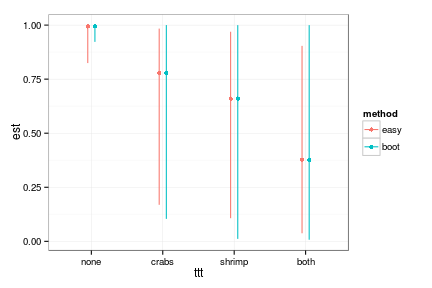The confidence intervals from the “easy” approach and the bootstrap approach are similar in this case. The bootstrap CIs are sometimes narrower (the lower CI for the predation probability of undefended corals is higher for the bootstrap than the “easy” CI) and sometimes larger (the upper bootstrap CIs for all of the other treatments extends all the way up to 1.0).

## Gopher tortoise

The data are from Ozgul et al. 2009s, Upper respiratory tract disease, force of infection, and effects on survival of gopher tortoises. Ecological Applications 19(3), 786-798.

### Data

load("gopherdat2.RData")
(gplot1 <- ggplot(Gdat,aes(x=prev,y=1+shells/Area))+
stat_sum(aes(colour=factor(year),
size=factor(..n..)))+
scale_size_discrete(range=c(3,6),name="overlap")+
scale_y_log10()+
scale_colour_discrete(name="year")+
geom_line(aes(group=Site),alpha=0.2)+
labs(x="Seroprevalence",y="1+shells/Area (log scale)"))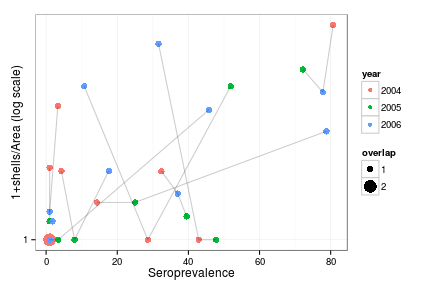There does indeed seem to be a general upward trend in shell density as a function of seroprevalence, but the rest of the plot is pretty messy. Lines connect observations from the same site (in an earlier version I used geom_text(aes(label=Site)) to label the points by site: this is useful for diagnostic purposes, but ugly).

### Fitting

#### glmer

Fit basic model with a log-linear effect of prevalence, an offset of log(Area) (to make the expected number of shells proportional to Area), year as a fixed categorical effect, and Site as a random effect.

gmod_lme4_L <- glmer(shells~prev+offset(log(Area))+factor(year)+(1|Site),
family=poisson,data=Gdat,
control=glmerControl(optimizer="bobyqa",

summary(gmod_lme4_L)

## Generalized linear mixed model fit by maximum likelihood (Laplace
##   Approximation) [glmerMod]
##  Family: poisson  ( log )
## Formula: shells ~ prev + offset(log(Area)) + factor(year) + (1 | Site)
##    Data: Gdat
## Control:
## glmerControl(optimizer = "bobyqa", check.conv.grad = .makeCC("warning",
##     0.05))
##
##      AIC      BIC   logLik deviance df.resid
##     86.3     93.3    -38.2     76.3       25
##
## Scaled residuals:
##    Min     1Q Median     3Q    Max
## -1.015 -0.625 -0.373  0.449  2.459
##
## Random effects:
##  Groups Name        Variance Std.Dev.
##  Site   (Intercept) 0        0
## Number of obs: 30, groups:  Site, 10
##
## Fixed effects:
##                  Estimate Std. Error z value Pr(>|z|)
## (Intercept)      -3.19732    0.27850  -11.48  < 2e-16 ***
## prev              0.02113    0.00431    4.90  9.4e-07 ***
## factor(year)2005 -0.64486    0.35639   -1.81     0.07 .
## factor(year)2006 -0.42876    0.31301   -1.37     0.17
## ---
## Signif. codes:  0 '***' 0.001 '**' 0.01 '*' 0.05 '.' 0.1 ' ' 1
##
## Correlation of Fixed Effects:
##             (Intr) prev   f()2005
## prev        -0.663
## fctr(y)2005 -0.464  0.040
## fctr(y)2006 -0.407 -0.138  0.384


This initial summary shows that the site-level variance is exactly zero (corresponding to pooling our data/ignoring the effect of Site: if you want to inspect this component of the summary by itself, you can specify VarCorr(gmod_lme4_L)). For comparison, we can also explicitly fit (non-mixed) generalized linear models with complete pooling and fixed effects of Site, respectively:

gmod_glm <- glm(shells~prev+offset(log(Area))+factor(year),
family=poisson,data=Gdat)
gmod_glm2 <- glm(shells~prev+offset(log(Area))+factor(year)+factor(Site),
family=poisson,data=Gdat)


Try the fit with Gauss-Hermite quadrature for comparison (perhaps GHQ will estimate a non-zero variance?)

gmod_lme4_agq <- update(gmod_lme4_L,nAGQ=10)


No:

VarCorr(gmod_lme4_agq)

##  Groups Name        Std.Dev.
##  Site   (Intercept) 0

AICtab(gmod_glm,gmod_glm2,gmod_lme4_L,logLik=TRUE)

##             dLogLik dAIC df
## gmod_glm     0.0     0.0 4
## gmod_lme4_L  0.0     2.0 5
## gmod_glm2    3.9    10.2 13


(At present lme4 computes log-likelihood/AIC values for models fitted with GHQ that are not comparable with glm() fits or glmer Laplace fits, so we skip gmod_lme4_agq in this summary.)

The pooled glm() and glmer() fits have identical log-likelihoods, as expected (when the random-effects variance collapses to 0, glmer() is essentially fitting a pooled model): the glmer() fit is AIC-penalized for an additional parameter (the among-site variance). The fixed-effect glm() fit has a slightly better log-likelihood, but not enough to make up for 9 additional Site effect parameters.

Another option in this case is to use bglmer from the blme package, which sets a weak prior on the variance to push it away from zero:

gmod_blmer_L <- bglmer(shells~prev+offset(log(Area))+factor(year)+(1|Site),
family=poisson,data=Gdat)

gmod_blmer_prof <- confint(gmod_blmer_L)

gmod_blmer_bootCI <- confint(gmod_blmer_L,method="boot")


Now we do estimate a positive (but small) among-site variance:

VarCorr(gmod_blmer_L)

##  Groups Name        Std.Dev.
##  Site   (Intercept) 0.301


Check for overdispersion: you can do this by hand by computing sum(residuals(gmod_lme4_L,"pearson")^2)), but the gof() function from the aods3 package is a handy shortcut (it computes overdispersion based on the deviance (D below) and Pearson residuals (X2): when they disagree, use the latter:

gof(gmod_lme4_L)

## D  = 76.34, df = 25, P(>D) = 4.23e-07
## X2 = 22.14, df = 25, P(>X2) = 0.6278


The sum of squared Pearson residuals is less than the residual degrees of freedom, so the response is actually underdispersed.

Just for comparison's sake, we'll fit a model with an observation-level random effect (logistic-normal model) anyway:

Gdat$obs <- factor(seq(nrow(Gdat))) gmod_lme4_L_od <- glmer(shells~prev+offset(log(Area))+factor(year)+(1|Site)+(1|obs), family=poisson,data=Gdat)  #### glmmADMB As before, the only thing we have to change for glmmADMB is to specify the family in quotation marks (family="poisson"): gmod_gA_L <- glmmadmb(shells~prev+offset(log(Area))+factor(year)+(1|Site), family="poisson",data=Gdat)  If we want, we have the alternative of fitting a negative-binomial model, either “type 1” ($$\text{Var} = \phi \mu$$) or “type 2” ($$\text{Var} = \mu (1 + \mu/k)$$): gmod_gA_L_NB2 <- glmmadmb(shells~prev+offset(log(Area))+factor(year)+(1|Site), family="nbinom",data=Gdat) gmod_gA_L_NB1 <- glmmadmb(shells~prev+offset(log(Area))+factor(year)+(1|Site), family="nbinom1",data=Gdat)  Unsurprisingly (since we know the data are underdispersed), the negative binomial model fits don't decrease the AIC: AICtab(gmod_gA_L,gmod_gA_L_NB1,gmod_gA_L_NB2)  ## dAIC df ## gmod_gA_L 0.0 5 ## gmod_gA_L_NB1 2.0 6 ## gmod_gA_L_NB2 2.1 6  #### MCMCglmm As in the previous example, it turns out we have to run MCMCglmm chains for longer, and specify reasonably strong priors, in order to get reasonable results. Offsets have to be set “by hand” by placing a strong prior on a coefficient of 1 for the offset term; the rest of the fixed-effect parameters get the usual mean of 0 and very large variance. B.prior <- list(V=diag(5)*1e7, mu=c(0,0,1,0,0)) B.prior$V[3,3] <- 1e-7  ## replace log(area) (offset) variance


For the variances, after finding that the default priors were too weak (see below), I initially used priors of the form list(V=5, nu=5) to specify fairly strongly that the variance could not be near zero, e.g.:prior.g <- list(B=B.prior,
R=list(V=1, nu=0.002),
G=list(G1=list(V=1, nu=1, alpha.mu=0, alpha.V=1000)))


Run with default parameters:

gmod_MG0 <- MCMCglmm(shells~prev+log(Area)+factor(year),
random=~Site,
family="poisson",data=Gdat,verbose=FALSE,
prior=list(B=B.prior))


Run for longer:

gmod_MG <- MCMCglmm(shells~prev+log(Area)+factor(year),
random=~Site,
family="poisson",data=Gdat,verbose=FALSE,
prior=list(B=B.prior),
nitt=1e5+3e3,thin=1e2)


Stronger/better priors (see above):

gmod_MG2 <- MCMCglmm(shells~prev+log(Area)+factor(year),
random=~Site,
prior=prior.g,
family="poisson",data=Gdat,verbose=FALSE,
nitt=1e5+3e3,thin=1e2)

## Using type as id variables
## Using type as id variables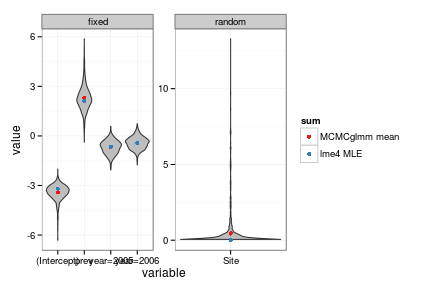### Diagnostics

The standard diagnostic plot for the glmer fits is a little better than in the binary case, but not much:

plot(gmod_lme4_L)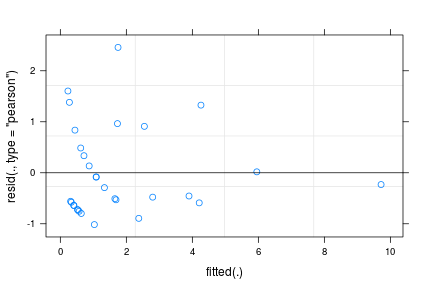plot(gmod_lme4_L,Site~resid(.))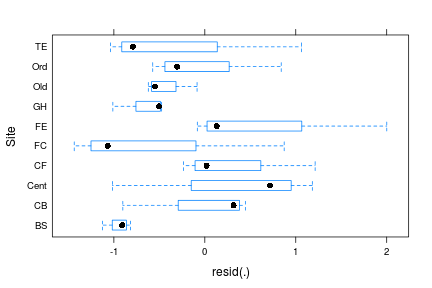However, it turns out that those boxplots are really only representing three values per site (i.e., site $$\times$$ year combinations): we can see this if we use ggplot to overlay points on the boxplots. While we're at it, we might as well reorder the Site factor to be in order of mean residuals. (WARNING: if you have loaded the scapeMCMC package to get prettier MCMC diagnostic plots such as plotTrace, you have also incidentally loaded the reorder.factor function from the gdata package, which changes the default behaviour of the reorder function, and there is a subtle bug in it as well. You need to make sure to specify FUN=mean and sort=sort explicitly to make it work …)

ff <- fortify(gmod_lme4_L)
ff <- transform(ff,Site=reorder(Site,X=.resid,FUN=mean,sort=sort))
ggplot(ff,aes(x=Site,y=.resid))+geom_boxplot()+
geom_point(size=4,alpha=0.5)+
coord_flip()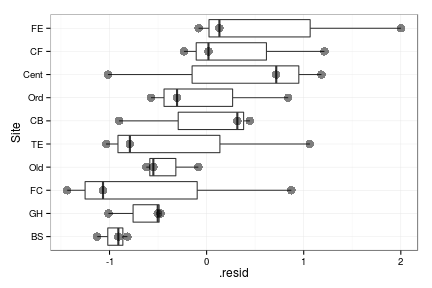There seems to be a fair amount of among-site variation, but apparently (at least according to glmer) this amount of among-site variation is still consistent with Poisson variation among otherwise identical sites …

We have already checked for overdispersion, above, and found the residuals to be under- rather than overdispersed.

We can do posterior predictive simulations to test whether the model is behaving like the data in other ways. For example, looking at the distributions of the numbers of zero-shell outcomes in simulated data:

sims <- simulate(gmod_lme4_L,nsim=1000)
nzeros <- colSums(sims==0)
par(las=1,bty="l")
plot(pt <- prop.table(table(nzeros)),
ylab="Probability")
(obszero <- sum(Gdat$shells==0))  ##  9  points(obszero,0.13,col="red",pch=16,cex=2)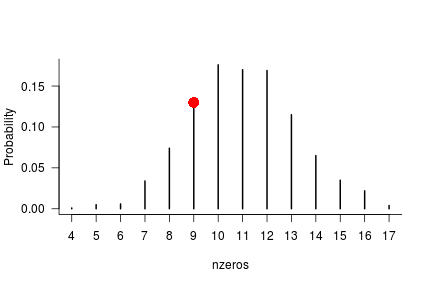A two-sided $$p$$-value, if we felt one were necessary: sum(pt[names(pt) %in% c(4:9,13:18)])  ##  0.485  We conclude that the model is doing a good job matching this characteristic of the data (i.e., we can't reject the null hypothesis that it's doing a good job …) We can easily do this for other characteristics of the data that we were interested in, such as among-site variance. In this case we will use the glm() fit to simulate and re-fit, since it's much faster than glmer() and suitable for this task: sims2 <- simulate(gmod_glm,nsim=1000) vfun <- function(x) { m_new <- update(gmod_glm,data=transform(Gdat,shells=x)) Gdat$.resid <- residuals(m_new,"pearson")
sitemeans <- ddply(Gdat,"Site",summarise,mresid=mean(.resid))
var(sitemeans$mresid) } vdist <- sapply(sims2,vfun)  Gdat$.glmresid <- residuals(gmod_glm,"pearson")
obs_sitemeans <- ddply(Gdat,"Site",summarise,mresid=mean(.glmresid))
obs_sitevar <- var(obs_sitemeans$mresid) par(las=1,bty="l") hist(vdist,breaks=30,col="gray",freq=FALSE,main="", xlab="Among-site variance in residuals") par(xpd=NA) ## prevent point getting cut off at top of plot points(obs_sitevar,3.1,col="red",pch=16,cex=2)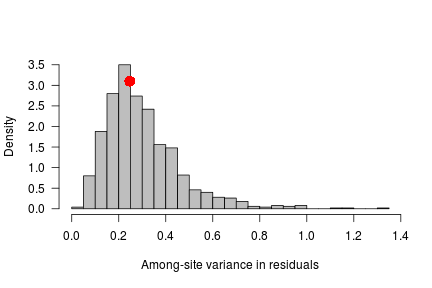The observed among-site variance is almost exactly what we would expect from Poisson variation with no true site-to-site variation. #### MCMCglmm We write a small utility function to automate the process of combining the fixed-effect (Sol) and variance-covariance (VCV) parameter chains and making the trace plot: tfun <- function(mod) { plotTrace(as.mcmc(cbind(mod$Sol,mod$VCV))) }  As suggested above, the default results are again bad. tfun(gmod_MG0)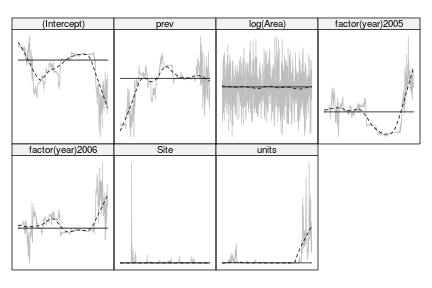Running for longer doesn't help that much: tfun(gmod_MG)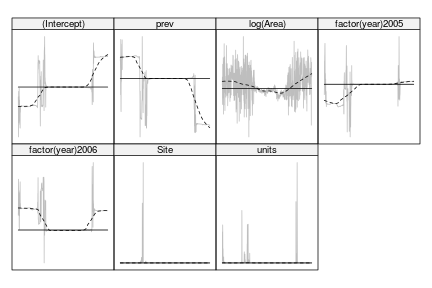Looks OK with the improved priors, though: tfun(gmod_MG2)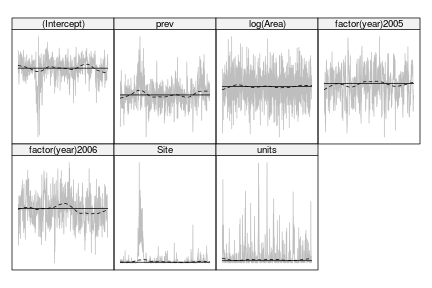We can again look at the variance parameters on the log scale to decide that they're not too bad: vcChain <- log10(gmod_MG2$VCV)
plotTrace(vcChain)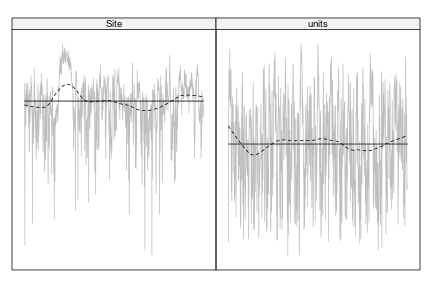### Inference

Computing confidence intervals and comparing estimates and CIs across the range of estimation and inference approaches:

gmod_CIwald <- confint(gmod_lme4_L,method="Wald")
gmod_CIprof <- confint(gmod_lme4_L,quiet=TRUE)
gmod_CIboot <- confint(gmod_lme4_L,method="boot",quiet=TRUE)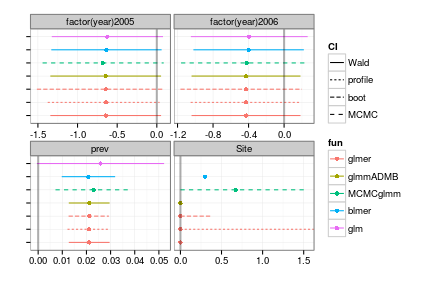I've plotted each of the parameters in a separate facet because their scales are somewhat different (it might also be worth scaling prev by its standard deviation in order to make comparisons, as suggested by Schielzeth 2010, but the Site variable would still differ somewhat).

• the year effects (which are of least interest) are fairly consistent among all methods, although MCMCglmm interprets the year-2005 parameter (i.e., the difference between 2004 and 2005) as being significantly different from zero.
• the effect of prevalence (which is of most interest) is similarly consistent, with an estimated effect of around 2% increase in shells per percentage seroprevalence. The blmer result, which forces a slightly positive among-site variance, has a slightly wider confidence interval; MCMCglmm wider still; and the fixed-effect model (i.e., glm with Site included as a factor) has a quite wide confidence interval – the latter is not surprising, as this model is quite overfitted (13 parameters for only 30 observations).
• The estimated among-Site standard deviations are mostly zero (except for MCMCglmm and blmer), but the confidence intervals vary widely; profile confidence intervals are the most conservative, followed by MCMCglmm and parametric bootstrap (it's not so easy to get the Wald confidence intervals on the random effect variances from the other models).

### Prediction

What if we want to re-plot our original data with predictions about the effect of seroprevalence overlaid?

g_pframe <- cbind(expand.grid(year=2004:2006,prev=0:80),Area=1)
g_pred <- predict(gmod_lme4_L,newdata=g_pframe,re.form=NA,
type="response")

g_predCI <- easyPredCI(gmod_lme4_L,newdata=g_pframe)

set.seed(101)
g_bb <- bootMer(gmod_lme4_L,
FUN=function(x)
predict(x,re.form=NA,newdata=g_pframe,
type="response"),
nsim=400)

gpredboot1.CI <- t(sapply(1:nrow(g_pframe),
function(i)
boot.ci(g_bb,type="perc",index=i)$percent[4:5])) ## or: t(apply(bb$t,2,quantile,c(0.025,0.975),na.rm=TRUE))

g_pframe2 <- cbind(g_pframe,shells=g_pred,g_predCI,
setNames(as.data.frame(gpredboot1.CI),
c("lwr_boot","upr_boot")))
gplot_pred1 <- gplot1 +
geom_line(data=g_pframe2,aes(colour=factor(year)))+
geom_ribbon(data=g_pframe2,
aes(group=factor(year),
ymin=1+lwr,ymax=1+upr),
alpha=0.1)
gplot_pred2 <- gplot1 +
geom_line(data=g_pframe2,aes(colour=factor(year)))+
geom_ribbon(data=g_pframe2,
aes(group=factor(year),
ymin=1+lwr_boot,ymax=1+upr_boot),
alpha=0.1)


The confidence intervals from the parametric bootstrap (right-hand plot) are quite similar:

grid.arrange(gplot_pred1,gplot_pred2,nrow=1)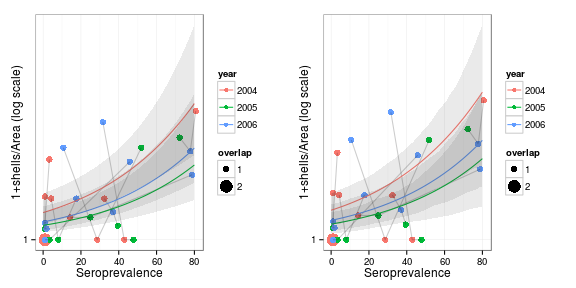## Grouse ticks

These data are those used in Elston et al., “Analysis of aggregation, a worked example: numbers of ticks on red grouse chicks”“ Parasitology, 122(5):563-569, 2001 (kindly donated by Robert Moss via David Elston).

tickdata = read.table("Elston2001_tickdata.txt",header=TRUE,
colClasses=c("factor","numeric","factor","numeric","factor","factor"))

ggplot(tickdata,aes(x=HEIGHT,y=1+TICKS,colour=YEAR))+
stat_sum(aes(size=..n..),alpha=0.7)+
scale_y_log10()+
scale_size_continuous(breaks=c(2,6,10),range=c(2,7))+
geom_smooth(method="glm",family=quasipoisson)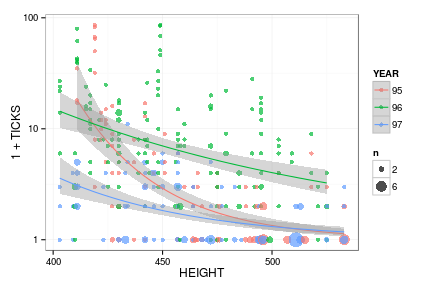The quasi-Poisson GLM fits shown here ignore the grouping structure, but are useful for structuring the data (although the HEIGHT effect is of secondary interest here – we're mostly interested in the relative variances at the individual, brood, and location levels).

Center the height data (as recommended by Gelman and Hill 2006, Schielzeth 2010) otherwise we will have trouble with both optimization- and MCMC-based approaches.

tickdata <- transform(tickdata,cHEIGHT=HEIGHT-mean(HEIGHT))


Because INDEX, BROOD, and LOCATION are uniquely labeled, we can specify the random effect as either (1|LOCATION)+(1|BROOD)+(1|INDEX) or (1|LOCATION/BROOD/INDEX), although the latter might be clearer:

tmod_lme4_L <- glmer(TICKS~YEAR+cHEIGHT+(1|LOCATION/BROOD/INDEX),
family="poisson",data=tickdata,
control=glmerControl(optimizer="bobyqa",


(I switched the optimizer used and increased the convergence-checking tolerance slightly here: hopefully this will be unnecessary in future versions of lme4.)

print(summary(tmod_lme4_L),corr=FALSE)

## Generalized linear mixed model fit by maximum likelihood (Laplace
##   Approximation) [glmerMod]
##  Family: poisson  ( log )
## Formula: TICKS ~ YEAR + cHEIGHT + (1 | LOCATION/BROOD/INDEX)
##    Data: tickdata
## Control:
## glmerControl(optimizer = "bobyqa", check.conv.grad = .makeCC("warning",
##     0.002))
##
##      AIC      BIC   logLik deviance df.resid
##   1794.5   1822.5   -890.3   1780.5      396
##
## Scaled residuals:
##    Min     1Q Median     3Q    Max
## -1.612 -0.554 -0.149  0.285  2.443
##
## Random effects:
##  Groups                 Name        Variance Std.Dev.
##  INDEX:(BROOD:LOCATION) (Intercept) 0.293    0.542
##  BROOD:LOCATION         (Intercept) 0.563    0.750
##  LOCATION               (Intercept) 0.280    0.529
## Number of obs: 403, groups:  INDEX:(BROOD:LOCATION), 403
## BROOD:LOCATION, 118 LOCATION, 63
##
## Fixed effects:
##             Estimate Std. Error z value Pr(>|z|)
## (Intercept)  0.37279    0.19637    1.90   0.0576 .
## YEAR96       1.18040    0.23812    4.96  7.2e-07 ***
## YEAR97      -0.97869    0.26278   -3.72   0.0002 ***
## cHEIGHT     -0.02376    0.00344   -6.91  4.8e-12 ***
## ---
## Signif. codes:  0 '***' 0.001 '**' 0.01 '*' 0.05 '.' 0.1 ' ' 1


This all looks reasonable; centering the height has given us a sensible intercept (otherwise it would correspond, ridiculously, to the expected number of ticks per grouse at sea level), the other parameters are sensible, and the variance is approximately equally divided between random-effects levels (when we work with variance decomposition it makes sense to interpret the random effects on the variance rather than the standard deviation scale).

tmod_gA_L <- glmmadmb(TICKS~YEAR+cHEIGHT+(1|LOCATION/BROOD/INDEX),
family="poisson",data=tickdata)


Since MCMCglmm automatically adds an observation-level random effect, we specify only BROOD and LOCATION, leaving out INDEX:

tmod_MG <- MCMCglmm(TICKS~cHEIGHT+YEAR,
random=~BROOD+LOCATION,
family="poisson",data=tickdata,
verbose=FALSE)


Once again we will need to try this with an informative prior. I initially specified (nu=5,V=1) for both the BROOD and LOCATION levels, but Hadfield suggests expanded parameters instead:

prior.t <- list(R=list(nu=0.002,V=1),
G=list(G1=list(V=1, nu=1, alpha.mu=0, alpha.V=1000),
G2=list(V=1, nu=1, alpha.mu=0, alpha.V=1000)))


The names of G-elements are ignored; it is their order that matters. Consequently, although the prior elements are named BROOD and LOCATION, because they appear in reverse order to their specification in the random formula the "BROOD” prior is actually associated with the LOCATION effect variance.

tmod_MG2 <- MCMCglmm(TICKS~cHEIGHT+YEAR,
random=~BROOD+LOCATION,
prior=prior.t,
family="poisson",data=tickdata,
verbose=FALSE)


Comparing results (just the random effects):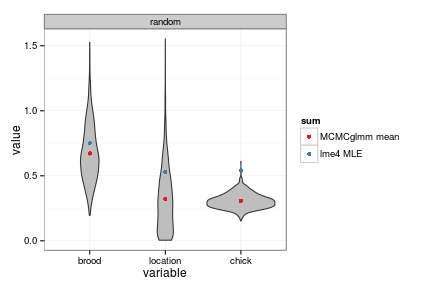For comparison, and because Elston et al. originally used penalized quasi-likelihood to fit their models, we use MASS::glmmPQL to fit the model. We don't include INDEX in this model either, because glmmPQL also includes a residual error term by default.

tmod_PQL <- glmmPQL(TICKS~cHEIGHT+YEAR,
random=~1|LOCATION/BROOD,
family="poisson",data=tickdata,
verbose=FALSE)


### Diagnostics

The diagnostic plot is clearer if we plot the fitted values on the log scale:

plot(tmod_lme4_L,residuals(.) ~log(fitted(.)))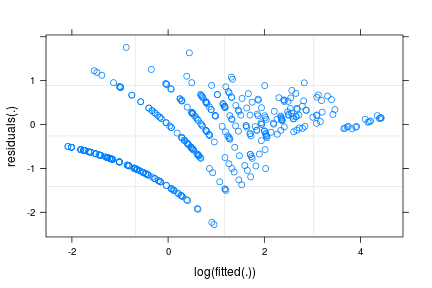ggplot(fortify(.)) puts the fitted values on the log scale by default. Here is a scale-location plot, which shows some tendency of the variance of the residuals to decrease with the mean:

ggplot(fortify(tmod_lme4_L),
aes(x=.fitted,y=sqrt(abs(.scresid))))+geom_point()+
geom_smooth(colour="red",alpha=0.3)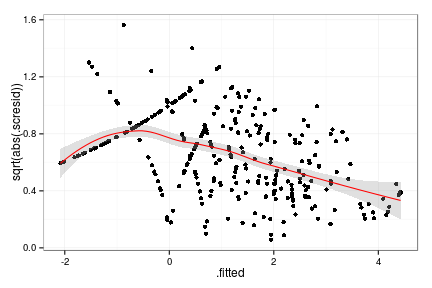We can look at the residuals grouped by location:

plot(tmod_lme4_L,LOCATION~resid(.,type="pearson"))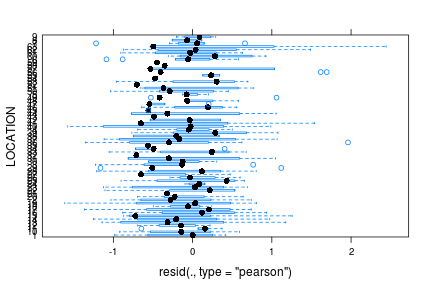ggplot makes it a little bit easier to re-order the locations by mean residual:

ff <- fortify(tmod_lme4_L)
ff <- transform(ff,LOCATION=reorder(LOCATION,.resid,fun=MEAN,sort=sort))
ggplot(ff, aes(x=LOCATION,y=.resid))+geom_boxplot(fill="gray")+coord_flip()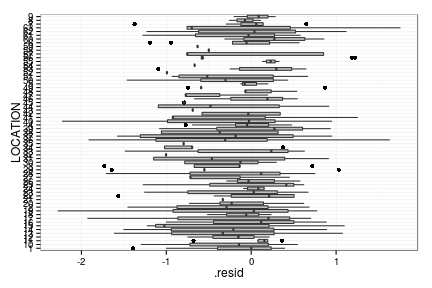We can also look at the conditional modes:

dd <- dotplot(ranef(tmod_lme4_L,condVar=TRUE))
do.call(grid.arrange,c(dd,list(nrow=1)))